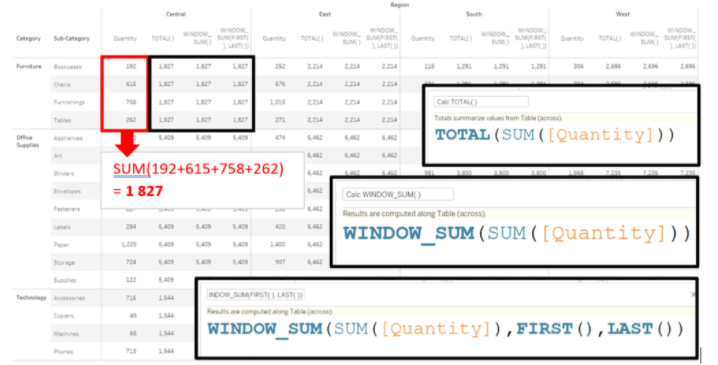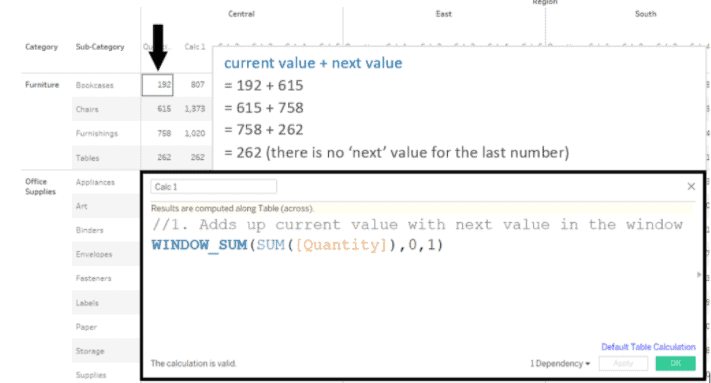# What is the difference between Tableau functions TOTAL() and WINDOW_SUM()?

• Tableau FAQs
• December 8, 2018

In this post, we review the difference between Tableau functions TOTAL() and WINDOW_SUM().

They are both Table Calculation functions. They both sum up values that are in your view.

• TOTAL( ) will always calculate the sum of all values in your window
• WINDOW_SUM( ) gives you more control over which values to include in your sum.

Both calculations will do the same thing if you do not set any values for where the WINDOW_SUM( ) function should start and end, or if you set it to start and end at the first and last values in the window.Notice, the syntax for the WINDOW_SUM( ) function allows you to select a start and endpoint anywhere between the first and last value in the window. Here are some examples of what you can do with that:## Related Posts

### Can you create a process flowchart normally built in a to...

• Tableau FAQs
• December 11, 2018

### How do I create a formula for only the last month of data...

• Tableau FAQs
• December 11, 2018